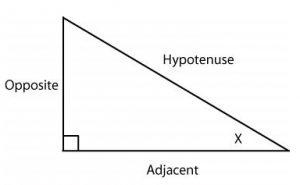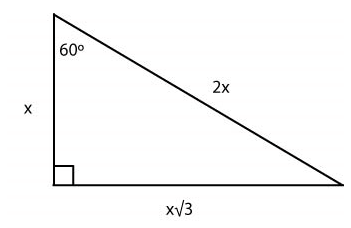# ACT Math: SOHCAHTOA and Trigonometry Graphing

A lot of people get intimidated when they hear the word “trigonometry.” And while it may dishearten you to find out that it’s tested on the ACT Math test, there’s really no need to worry. All you need to know is your right triangle, and it’ll be cake.

### Sine, Cosine, and Tangent

The standard way of learning the right triangle relationships and their angles, is the mnemonic SOHCAHTOA. But before we get into that, let’s learn the basics of trigonometry.
You’ll see the words “sine”, “cosine”, and “tangent” quite a bit when dealing with trigonometry, so it’s good to know their definitions.We see that for angle X, there are the three sides relative to the angle. The hypotenuse, the adjacent side, and the opposite side. Let’s say we want to find sinX, we take the length of the opposite side, and put it over the length of the hypotenuse.
This is where our trusted mnemonic comes in. SOHCAHTOA. To break it down, Sine = Oppostie/Hypotenuse, Cosine = Adjacent/Hypotenuse, Tangent = Opposite/Adjacent (or, SOHCAHTOA)
Let’s try a practice triangle, to get this idea down.Using the SOHCAHTOA method, we can find the values of sin60, cos60 and tan60.
Sin60 = √3/2 cos60 = 1/2 and tan60 = √3/1
Now that we have this concept down, the ACT might also ask you to figure out the information by working backwards. However, while this may seem daunting, knowing what we do about SOHCAHTOA, it should be very simple.

### Graphing Trigonometry Functions

In order to graph trig functions, you need to understand some Trig basics. Sine, cosine, and tangent are the three main trig identities. They are usually graphed and expressed in degrees, but you may also see them expressed in radians. There are 2π radians in one circle. Each point on a circle corresponds to a certain number of radians. To convert degrees to radians, simply multiply by π/180.Sine and cosine both have standard graphs that you need to memorize. The standard equation for sine looks like this:  y = sin x. The “period” of the wave is how long it takes the curve to reach its beginning point again. The coefficient in front of “sin” (here 1), is called the amplitude. It effects how high and how low the wave reaches vertically. If that coefficient changes, then the height changes. For example, y = 3 sin x, would show a curve that reaches +3 on the y-axis and extends down to -3 on the y-axis.Cosine also has a standard equation. It looks like: y = cos x. For the graph of cosine, notice how it begins at its highest y-value and descends, whereas sine begins at the origin. Cosine and sine have the same period of 2π. Questions involving trig graphs will likely require you to match given equations with graphs, or interpret the meaning of certain graphs, such as in a question like this: What is the smallest positive value for x where y = cos 2x ? The difference between y = cos x and y = cos 2x is that the coefficient in front of x is halving the period, so it will now take just one pi to complete its cycle. The smallest x-value for cosine usually occurs at π/2. For the new graph, it will occur at π/4, which is ½ of π/2. Let’s try a practice question:

We know that the coefficient in front of sine changes the amplitude, so A and B can quickly be eliminated, since 4 multiplies the amplitude by 4. Just like a linear equation, adding to the end of an equation shifts a graph upwards. For example, the only difference between y = 8x and y = 8x + 7 is that the latter is 7 places higher on the y-axis. The answer is D.

Tags: